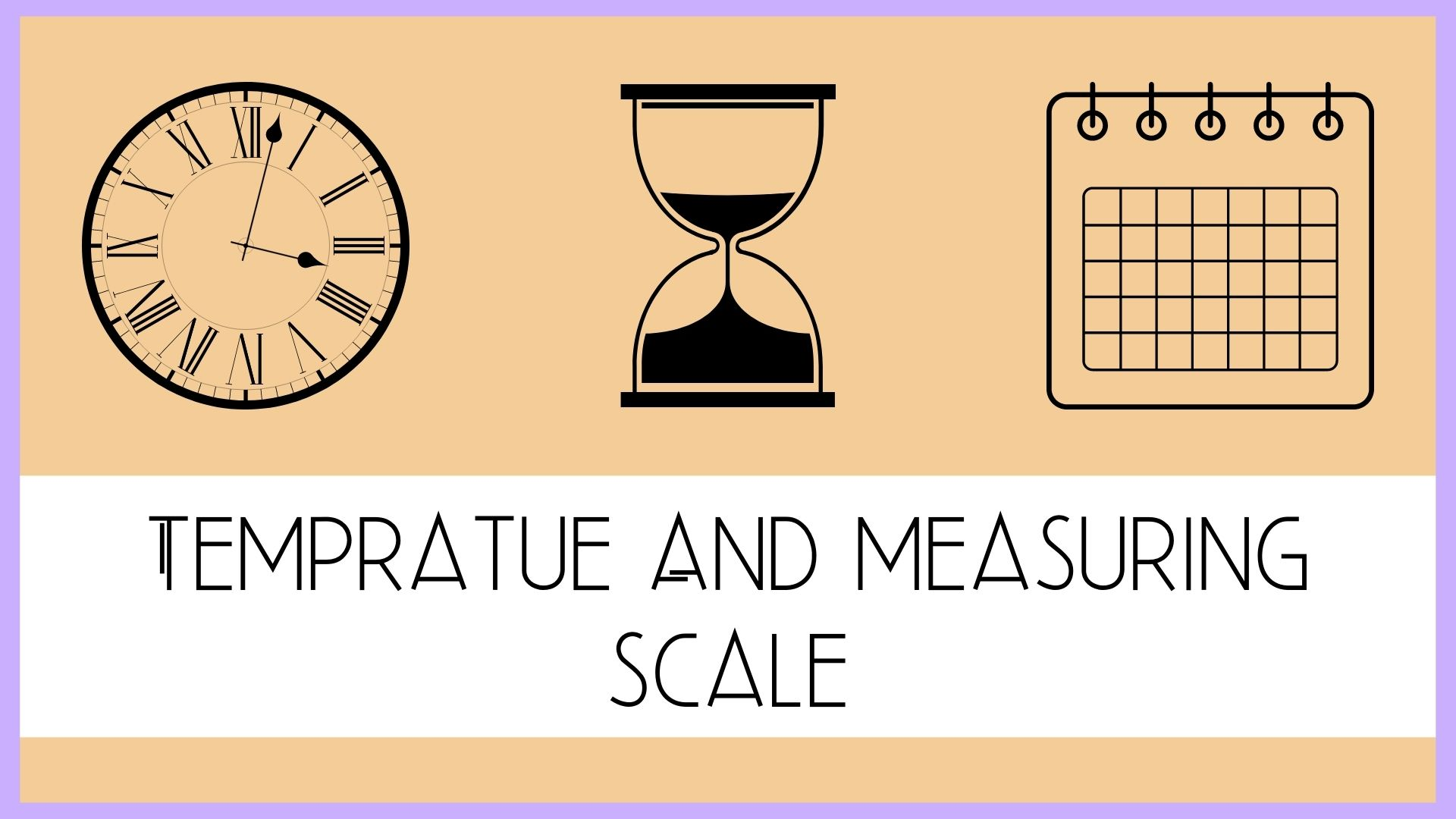# Know About Temperature and Its Measuring Scales

## Introduction to Temprature

It can be defined in many ways, as, “the degree or intensity of heat present in a substance or object, especially as expressed according to a comparative scale and shown by a thermometer or perceived by touch or the degree of the internal heat of a person’s body. So, there are three scales of temperature or we can say three lenses by which is measured in three different ways.

## Three Measuring Scales of Temperature

Three Measuring Scales are:

1. Fahrenheit scale
2. Celsius scale
3. Kelvin scale

Until the 1970s the Fahrenheit scale was in general common use in English-speaking countries; the Celsius, or centigrade, the scale was employed in most other countries and for scientific purposes worldwide.

Let’s move ahead to know more about three of the measuring scales.

## Fahrenheit Temperature scale

Fahrenheit scale is a scale based on 32 degrees for the freezing point of water and 212 degrees for the boiling point of water. The distance or interval of degrees between these two points is divided into 180 parts.

A German physicist Daniel Gabriel Fahrenheit in 18th century, at first took as the zero of his scale equal to the temperature of an ice-salt mixture and selected the value of 30 for the freezing point of water and 90 for the normal body temperature, respectively; these later were revised to 32 and 96, but the final scale required an adjustment and decided to 98.6 for the normal body temperature.

## Celsius Temperature Scale

Celsius scale is also called the centigrade temperature scale. This scale is based on 0 degrees for the freezing point of water and 100 degrees for the boiling point of water. It was invented in 1742 by the Swedish astronomer Anders Celsius. Celsius scale can be used both for routine and scientific purposes.

## Kelvin Temperature Scale

Kelvin scale is the base unit of thermodynamic temperature measurement in the International System (SI) of measurement. Moreover, it can be called the absolute temperature scale.

## Conversion of Temperature Scales

Scientists are often required to convert one temperature scale to another for different purposes. Fahrenheit scale is a standard scale for medical purposes especially for the measurement of human body temperature. On the other hand, the Celsius scale is used to measure the temperature of the climate and also for measuring the boiling and freezing point of water. Thus there are formulas of conversion of these scales which were generated by the relevant scientists. Every scale has its own formula for conversion.

You can also use a Converter Calculator based on technology to convert one temp scale to another. Indeed, these calculators are efficient enough to convert temperatures scales smartly, and quickly.

Anyhow, let’s have a look at the manual conversion methods of Temperature Scales.

### Celsius to Fahrenheit

Celsius to Fahrenheit: The conversion formula for a temperatures that is expressed on the Celsius (C) scale to its Fahrenheit (F) representation is: F = 9/5C + 32, OR F= C + 9/5 32

example:

0 degrees Celsius is equal to 32 degrees Fahrenheit:

0 °C = 32 °F

The temperature T in degrees Fahrenheit (°F) is equal to the temperature T in degrees Celsius (°C) times 9/5 plus 32:

T(°F) = T(°C) × 9/5 + 32

For example, If you are required to 20 degrees Celsius to degrees Fahrenheit then T(°F) = 20°C × 9/5 + 32 = 68 °F.

You may also calculate it by using Celsius to Fahrenheit calculator.

### Fahrenheit to Celcius

Fahrenheit to Celcius:  The Celsius scale is in general use wherever metric units have become accepted, and it is used in scientific work everywhere. The following formula can be used to convert temperatures from Fahrenheit ( F) scale to the Celsius (C) value: C = 5/9(F – 32).

Example:

0 degrees Fahrenheit is equal to -17.77778 degrees Celsius:

0 °F = -17.77778 °C

The T in degrees Celsius (°C) is equal to the T in degrees Fahrenheit (°F) minus 32, times 5/9:

T(°C) = (T(°F) – 32) × 5/9

Example:

Convert 68 degrees Fahrenheit to degrees Celsius:

T(°C) = (68°F – 32) × 5/9 = 20 °C

### Celsius to Kelvin

Celsius to Kelvin: Kelvin scale has been adopted as the international standard for scientific temperatures measurement. The Kelvin scale is related to the Celsius scale. The difference between the freezing and boiling points of water is 100 degrees in each so that the kelvin has the same magnitude as the degree Celsius.

The formula to convert the Celsius to Kelvin temperature scale is T(K) = T(°C) + 273.15.

Example:

The T in Kelvin (K) is equal to the T in degrees Celsius (°C) plus 273.15 :

T(K) = T(°C) + 273.15

Example:

Convert 10 degrees Celsius to Kelvin:

T(K) = 10°C + 273.15 = 283.15 K.

Uneeb Khan

Uneeb Khan CEO at marketgit.com. Have 3 years of experience in the websites field. Uneeb Khan is the premier and most trustworthy informer for technology, telecom, business, auto news games review in World. Read Also: Best Alternative APK Of Pikashow For Android Read Also: google web stories Read Also: Ines de Ramon Read Also: Car Accident Chiropractor in Denver Read Also: Sudden Drop in Website Traffic Read Also: All About Undertaker’s Wife Michelle McCool Read Also: December Global Holidays Also Read: MCU TV shows on Disney plus Read Also: traffic dropped due to Read Also: lola iolani momoa biography Read Also: Google site kit